CS 61B: Data Structures (Spring 2005)
Midterm II

### Solutions

Problem 1. (8 points) Quickies.

a. The worst-case time to find the second-smallest key in a 2-3-4 tree with n keys is Θ(log n).

b. Algorithm A runs in O(n log n) worst-case time, and Algorithm B runs in Ω(n2) worst-case time. It is not always true that Algorithm A takes less time than Algorithm B. There are at least two possible counterarguments (either of them worth full credit). First, on some inputs, Algorithm B might run much faster than its worst-case time. Second, the constants hidden in the asymptotic notation might be much smaller for Algorithm B than for Algorithm A, in which case Algorithm B might be much faster on small inputs.

c. The line System.out.println(e); is acceptable. It calls e.toString();, and prints the string returned. (Note that toString is defined on all Objects.)

d.e.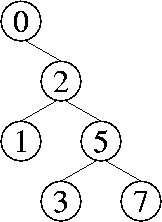f. Suppose that, through a sequence of operations, we create a tree with the keys 1 through n, where n is one less than a power of two. Because each operation always yields a tree with the minimum possible height, the tree must appear as follows.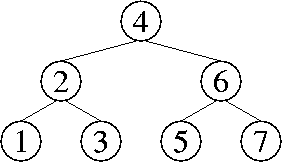Now, suppose you delete(1) and insert(n + 1). Because each operation always yields a tree with the minimum possible height, the tree must appear as follows.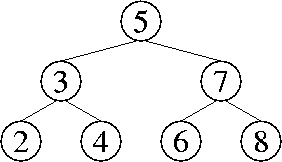Every key's parents and children have changed, so Θ(n) changes have been made to the tree. Therefore, either the delete or the insert operation took Ω(n) time.

g. The central tenet of Britney Theory is that celebrities play the archetypal roles in our lives that were once played by pantheistic gods.

Problem 2. (7 points) Trees.

a.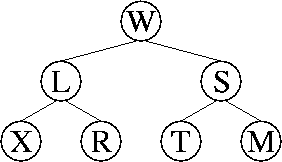b.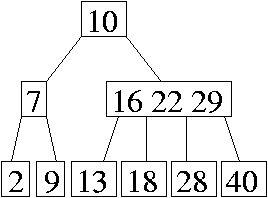c.

```
class BinaryTreeNode {
Entry entry;
BinaryTreeNode parent;
BinaryTreeNode leftChild, rightChild;

public BinaryTreeNode(Entry[] entries, int first, int last) {
parent = null;   leftChild = null;   rightChild = null;
int mid = ____(first + last) / 2_________________________;
entry = entries[mid];
if (mid > first) {
leftChild = new BinaryTreeNode(entries, __first___, __mid - 1_);
leftChild.parent = ____this___________________________;
}
if (mid < last) {
rightChild = new BinaryTreeNode(entries, __mid + 1_, __last____);
rightChild.parent = ____this__________________________;
}
}
}
```

Problem 3. (4 points) Asymptotic Analysis.

To prove that max{x, y} ∈ Θ(x + y), we have to do two proofs: one for the big-Oh bound, and one for the big-Ω bound.

max{x, y} ∈ O(x + y):

Choose c = 1, X = 0, Y = 0.
It is clear that for all x > 0 and all y > 0, x < x + y and y < x + y.
Therefore, for all x > X and all y > Y, max{x, y} < x + y, which confirms that max{x, y} ∈ O(x + y).

x + y ∈ O(max{x, y}):

Choose c = 2. (X and Y can have any values.)
There are two cases.
If xy, we see that x + y ≤ 2x = 2 max{x, y}.
If x < y, we see that x + y < 2y = 2 max{x, y}.
In either case, x + y ≤ 2 max{x, y}, which confirms that x + y ∈ O(max{x, y}).

Problem 4. (7 points) Reordering a Singly-Linked List.

a. ABDEFC

b. EBDFAC

c.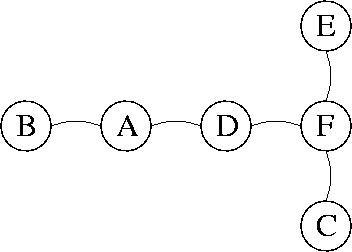d. Do a traversal of the binary search tree. (It doesn't matter which traversal, as long as it takes linear time.) When you visit a node, create an adjacency list for the node with up to three items: its parent (if it has one) and its children (if it has any).

Mail inquiries to cs61b@cory.eecs